Home  |  Contact
 Email: Password:
 Secreto Masonico

 What’s NewJoin Now
Message Board
Image Gallery
Files and Documents
Polls and Test
Member List
EL SECRETO DE LA INICIACIÓN
Procesos Secretos del Alma
Estructura Secreta del Ritual Masónico
Los extraños Ritos de Sangre
Cámara de Reflexiones

Tools

General: 33 TAMBIEN SALE DE LOS OCHO DIAS DE LA CREACION DIVINA
Choose another message boardPrevious subject  Next subjectReply Message 1 of 19 on the subject
 From: BARILOCHENSE6999  (Original message) Sent: 03/05/2015 17:22
GRAN PIRAMIDE DISEÑADA EN FUNCION A LA MANZANA DORADA (NUMERO DE ORO PHI=1.618033), AL NUMERO 4 (CUATRO FASES DE LA LUNA CADA SIETE DIAS O CUARTO MANDAMIENTO CON REFERENCIA AL SEPTIMO DIA) QUE TAMBIEN ES EL CUADRADO DE LA CUADRATURA, AL CIRCULO (POLIGONO DE INFINITO=NUMERO 8=OCTAVO DIA) QUE ES LA CONVERSION DEL AGUA EN VINO, EN EL MARCO A LAS BODAS DE CANA.

Squaring the Circle - The Great Pyramid
 The square represents the physical. The circle represents the spiritual. All sacred geometers have attempted the impossible: to square the circle (create a square who's perimeter is equal to the circumference of a circle.)   Here is the first of two valiant attempts: This squaring of the circle works with a right triangle that represents the apothem (ZY) - (a line drawn from the base of the center of one of the sides to top of the pyramid), down to the center of the base (ZE), and out to the point where the apothem touches the Earth (EY).The Great Pyramid of Egypt (Sphinx in foreground)Now let's look at this in 2D, from directly above. For the purpose of this exercise, the side (AB) of the base equals 2.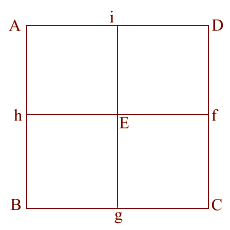(ABCD) is the base of the Great Pyramid. This is lettered similarly to the wire frame version (above). For the purpose of this exercise, the side (AB) of the base equals 2.Construct square (i JKD), thus creating double square (JKE f).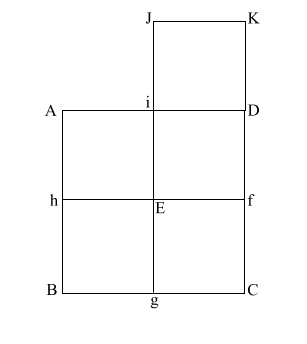Create diagonal (EK) which intersects (i D) at (l). iD = 1, therefore the diameter of the circle is also 1. (EK) = (5) = .618 + 1 + .618Put the point of your compass at (E) and extend it along the diagonal (EK) to point (m) where the circle intersects (EK), and draw the arc downward to intersect (KD f C) at (n). If (EK) = (5), and (l m/l D) and l i = .5, the diameter of this circle is 1.   This makes (E m) = .618 + 1, or 1.618. (E m) is the apothem.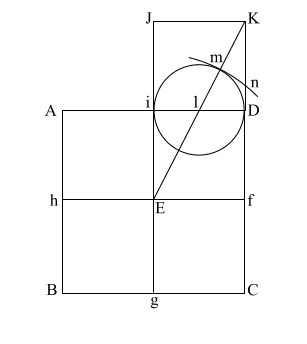Draw (E n) which intersects (A i l D ) at (o). Put compass point at (f) and extend it to (n). Again put your point at (E) and draw the circle which happens to have the radius (E o). (f n) is the height of the Great Pyramid. This circle comes remarkably close to having the same circumference as the perimeter of the base (ABCD).

Let's go back to the original right triangle (EYZ)
(EY) = .5

(YZ) = phi

(EZ) = (phi)EY = .5, The apothem is phi/1.618. This makes the 51 degree + degree angle.

Using a² + b² = c², this makes the height the square root of phi.

Squaring the Circle - The Earth & the Moon

 Create a square (ABCD) with (AB) = 11 Create diagonals (AC) and (BD) crossing at center point (E) Construct a circle which is tangent to square (ABCD) at f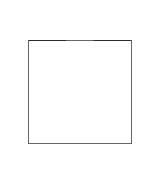Construct two 3 . 4 . 5 right triangles, with the 4 . 5 angles at (A) and (D). Connect the 5 . 3 angles creating square (abcd) with side (ab) = 3 {4 + 3 + 4 = 11, or side (AD) of square (ABCD)} Create diagonals (ac) and (bd) centering at (e) Create a circle that is tangent to square (abcd) at four places.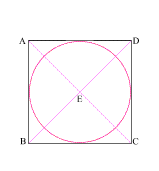Draw line (Ee) which intersects side (AD) at (F) (EF) = the radius of the larger circle and (eF) = the radius of the smaller circle The larger circle thus created is to the smaller circle as the moon is to the Earth! With your compass point at (E), create a circle with radius (Ee) This creates a circle whose circumference is equal to the perimeter of square (ABCD)!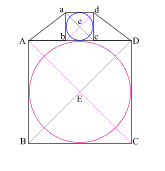The Math:

 1 (AB) = 11 (EF) = 1/2 of (AB) = 5.5 (ab) = 3 (eF) = 1.5 Therefore   5.5 + 1.5 = 7The circumference of a circle is equal to two times the radius (the diameter) times pi (3.1416). C= 14 x 3.1416 C= 43.9824 2 In Square (ABCD), (AB) = 11 The perimeter of a square is four times one side. 11 x 4 = 44 According to the Cambridge Encyclopedia, the equator radius of the Earth is 3963 miles. The equator radius of the Moon is 1080. The claim is that the smaller circle (in square abcd) is to the larger circle (in square ABCD) as the Moon is to the Earth.
 3 (EF) = 5.5 (F e) = 1.5 5.5 : 1.5 :: 3963 : 1080 5.5 / 1.5 = 3.66666 3963 / 1080 = 3.6694 - (if it had been 3960, it would have been exact!)
EL NUMERO DE ORO PHI=1.618033 SALE MATEMATICAMENTE DE LA UNION DE DOS CUADRADOS (FORMA DE NUMERO OCHO)NOTEN EN LA GRAFICA SUPERIOR, QUE ES EL NUMERO OCHO, EN LA PARTE SUPERIOR TENEMOS TRES LINEAS, AL IGUAL QUE EN LA PARTE INFERIOR. EN LA INTERSECCION, OSEA EL AGUJERO DE GUSANO, TENEMOS DOS LINEAS, QUE AL FINAL SON UNA. LO INCREIBLE QUE EN LA MISA CATOLICA CUMPLE ESTE PATRON EN EL CONTEXTO A LA SANTA CENA, CUANDO EL SACERDOTE HACE REFERENCIA AL PAN, SE TOCAN TRES VECES LA CAMPANA, LUEGO CON EL VINO, SE VUELVE A TOCAR TRES VECES LA CAMPANA Y AL FINAL SE TOCA, UNA, OSEA, INSISTO, LA INTERSECCION.FirstPrevious  2 to 4 of 19  NextLastReply Message 2 of 19 on the subject
 From: BARILOCHENSE6999 Sent: 05/05/2015 16:03

# Ocho

8
Cardinal Ocho
Ordinal Octavo, -a
Factorización
Sistemas de numeración
Romana VIII
Ática ΠΙΙΙ
Jónica η
China
China tradicional
Egipcia IIIIIIII
Armenia Ը
MayaCirílica И
De los Campos de Urnas ///
India
Sistema binario 1000
Sistema octal 10
Sistema hexadecimal 8
Como parámetro de una función
Función φ de Euler 4
Función divisor 4
Función de Möbius 0
Función de Mertens -2
 siete 8 nueve
Potencias de dos
 22 23 24
Lista de números
[editar datos en Wikidata]

El ocho (8) es el número natural que sigue al siete y precede al nueve.

## Características[editar]

• Es el número atómico del oxígeno.
• En el sistema anglosajón de mediciones, hay 8 onzas en una taza, 8 pintas en un galón y 8 furlongs en una milla.
• El 8 se representa con las 2 serpientes entrelazadas del caduceo, símbolo del equilibrio entre fuerzas antagónicas. También representa el eterno movimiento cósmico base de regeneración y de infinito. Es verticalidad formal del infinito.
• En la mística cosmogónica de la Edad Media el 8 correspondía al cielo de las estrellas fijas (Firmamento) y simbolizaba el perfeccionamiento de los influjos planetarios. 
• En la República Popular China, el 8 es un número que simboliza la buena suerte. 
Evolución de la grafía del número ocho en el sistema de numeración indoarábigo.

## Referencias[editar]

1. Volver arriba Diccionario de símbolos, de Juan Eduardo Cirlot.

 Reply Message 3 of 19 on the subject
 From: BARILOCHENSE6999 Sent: 05/05/2015 18:46Reply Message 4 of 19 on the subject
 From: BARILOCHENSE6999 Sent: 06/05/2015 16:48SOL-O-MON (BOAZ Y JACHIN) SOL Y LUNA   INTERSECCION ES EL HIJO (VESICA PISCIS= TEORIA DE CONJUNTOS)FirstPrevious  2 a 4 de 19  NextLastPrevious subject  Next subject©2021 - Gabitos - All rights reserved